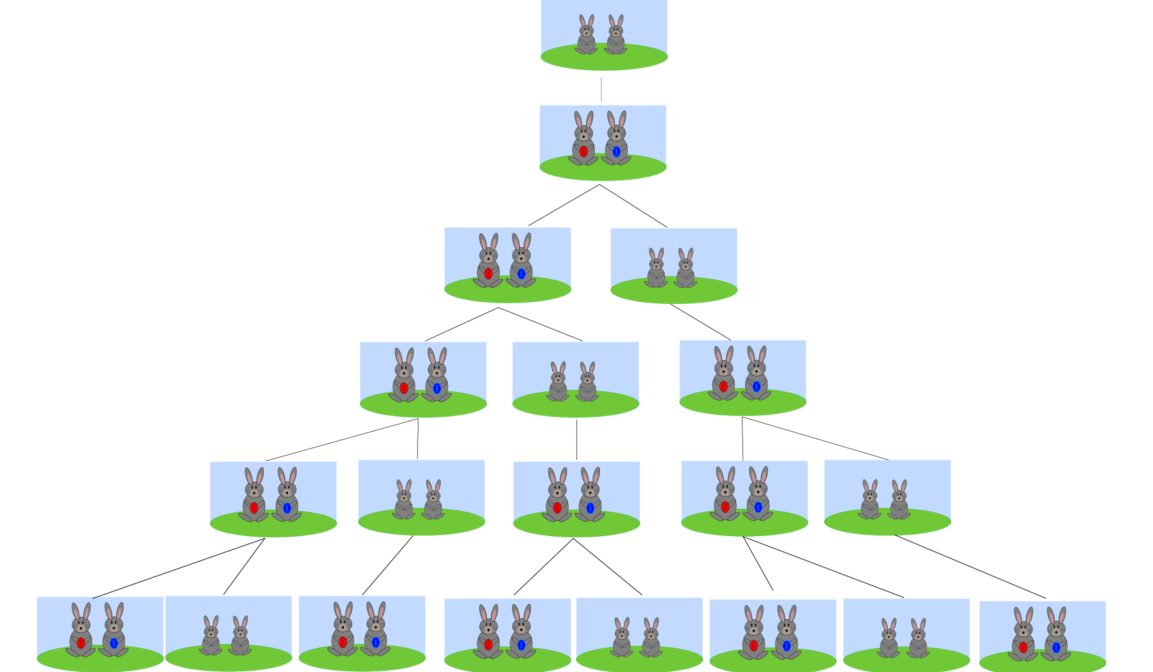#Rosalind: 兔子与递归

0
0
01. 云栖社区>
2. 博客>
3. 正文

## 问题描述## 结题思路#include <stdio.h>
#include <stdlib.h>

long int FIB(long int n, long int k)
{
if (n == 1 || n == 2){
return 1;
} else{
return FIB(n-1,k) + k * FIB(n-2, k);
}

}

int main(int argc, char *argv[])
{
if (argc != 3){
printf("USAGE: ./FIB n k \n");
return 1;
}
long int n = atoi(argv);
long int k = atoi(argv);
printf("month is %ld, %ld rabbit pairs per \n", n, k);
long total = FIB(n,k);
printf("The total number of rabbit is %ld \n", total);
return 0;

}


#include <stdio.h>
#include <stdlib.h>

int main(int argc, char *argv[])
{
if (argc != 2){
printf("USAGE: ./FIBv2 n k \n");
}

long int n = atoi(argv);
long int k = atoi(argv);

long int month[n-1];
int i = 0;
for (i = 0 ; i < n; i++){
if ( i == 0 || i == 1){
month[i] = 1;
} else{
month[i] = month[i-1] + k * month[i-2];
}
}

printf("After %ld month, there are %ld rabbits\n", n, month[n-1]);

return 0;
}


+ 关注